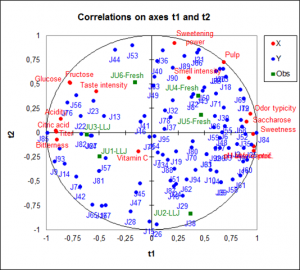Partial Least Squares regression (PLS) is often used when there are a lot of explanatory variables, possibly correlated. Available in Excel with XLSTAT.Partial Least Squares regression (PLS) is a quick, efficient and optimal regression method based on covariance. It is recommended in cases of regression where the number of explanatory variables is high, and where it is likely that the explanatory variables are correlated.

## What is Partial Least Squares regression

The idea behind the PLS regression is to create, starting from a table with n observations described by p variables, a set of h components with the hPLS1 and PLS2 algorithms

Some programs differentiate PLS1 from PLS2. PLS1 corresponds to the case where there is only one dependent variablePLS2 corresponds to the case where there are several dependent variables. The algorithms used by XLSTAT are such that the PLS1 is only a particular case of PLS2.

## Partial Least Squares regression model equations

In the case of the OLS and PCR methods, if models need to be computed for several dependent variables, the computation of the models is simply a loop on the columns of the dependent variables table Y. In the case of PLS regression, the covariance structure of Y also influences the computations.

The equation of the PLS regression model writes:

Y = ThC’h + Eh = XWh*C’h + Eh = XWh (P’hWh)-1 C’h + Eh

where Y is the matrix of the dependent variables, X is the matrix of the explanatory variables. Th, Ch, W*h , Wh and Ph, are the matrices generated by the PLS algorithm, and Eh is the matrix of the residuals.

The matrix B of the regression coefficients of Y on X, with h components generated by the PLS regression algorithm is given by:

B = Wh(P’hWh)-1C’hNote: the PLS regression leads to a linear model as the OLS and PCR do.

PLS regression results: Correlation, observations charts and biplots

A great advantage of PLS regression over classic regression are the available charts that describe the data structure. Thanks to the correlation and loading plots it is easy to study the relationship among the variables. It can be relationships among the explanatory variables or dependent variables, as well as between explanatory and dependent variables. The score plot gives information about sample proximity and dataset structure. The biplot gather all these information in one chart.

## Prediction with Partial Least Squares regression

PLS regression is also used to build predictive models. XLSTAT enable you to predict new samples' values.

## General remarks about PLS regression

The three methods – Partial Least Squere regression, Principal Componenet regression and Ordinary Least Squares regression - give the same results if the number of components obtained from the PCA (in PCR) or from the PLS regression is equal to the number of explanatory variables.

The components obtained from the PLS regression are built so that they explain as well as possible Y, while the components of the PCR are built to describe X as well as possible. The XLSTAT-PLS software allows partly compensating this drawback of the PCR by allowing the selection of the components that are the most correlated with Y.### analice sus datos con xlstat

prueba gratuita de 14 días

Incluido en